# Diagnosing Potential Predictability¶

This demo demonstrates climpred’s capabilities to diagnose areas containing potentially predictable variations from a control or reference alone without requiring multi-member, multi-initialization simulations. This notebook identifies the slow components of internal variability that indicate potential predictability. Here, we showcase a set of methods to show regions indicating probabilities for decadal predictability.

:

import warnings
%matplotlib inline
import climpred
warnings.filterwarnings("ignore")

:

# Sea surface temperature
varname='tos'


## Diagnostic Potential Predictability (DPP)¶

We can first use the [Resplandy 2015] and [Seferian 2018] method for computing the unbiased DPP by not chunking the time dimension.

:

# calculate DPP with m=10
DPP10 = climpred.stats.dpp(control3d, m=10, chunk=False)
# calculate a threshold by random shuffling (based on bootstrapping with replacement at 95% significance level)
threshold = climpred.bootstrap.dpp_threshold(control3d,
m=10,
chunk=False,
bootstrap=10,
sig=95)
# plot grid cells where DPP above threshold
DPP10.where(DPP10 > threshold).plot(yincrease=False, vmin=-0.1, vmax=0.6, cmap='viridis')

:

<matplotlib.collections.QuadMesh at 0x7fa3315bb908>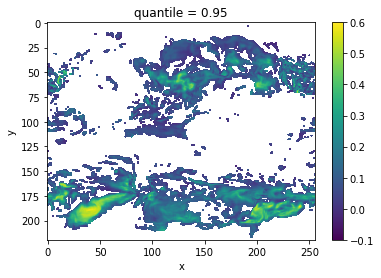Now, we can turn on chunking (the default for this function) to use the [Boer 2004] method.

:

# chunk = True signals the Boer 2004 method
DPP10 = climpred.stats.dpp(control3d, m=10, chunk=True)
threshold = climpred.bootstrap.dpp_threshold(control3d,
m=10,
chunk=True,
bootstrap=50,
sig=95)
DPP10.where(DPP10>0).plot(yincrease=False, vmin=-0.1, vmax=0.6, cmap='viridis')

:

<matplotlib.collections.QuadMesh at 0x7fa331c7e0b8>## Variance-Weighted Mean Period¶

A periodogram is computed based on a control simulation to extract the mean period of variations, which are weighted by the respective variance. Regions with a high mean period value indicate low-frequency variations with are potentially predictable [Branstator 2010].

:

vwmp = climpred.stats.varweighted_mean_period(control3d, dim='time')
threshold = climpred.bootstrap.varweighted_mean_period_threshold(control3d,
bootstrap=10)
vwmp.where(vwmp > threshold).plot(yincrease=False, robust=True)

:

<matplotlib.collections.QuadMesh at 0x7fa3131b7240>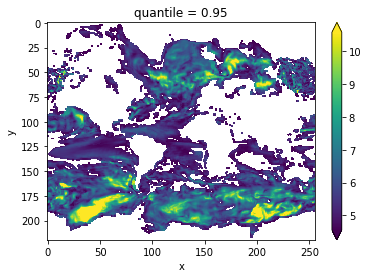## Lag-1 Autocorrelation¶

The lag-1 autocorrelation also indicates where slower modes of variability occur by identifying regions with high temporal correlation [vonStorch 1999].

:

# use climpred.bootstrap._bootstrap_func to wrap any stats function
threshold = climpred.bootstrap._bootstrap_func(climpred.stats.autocorr,control3d,'time',bootstrap=100)
corr_ef = climpred.stats.autocorr(control3d, dim='time')
corr_ef.where(corr_ef>threshold).plot(yincrease=False, robust=False)

:

<matplotlib.collections.QuadMesh at 0x7fa3213b8cc0>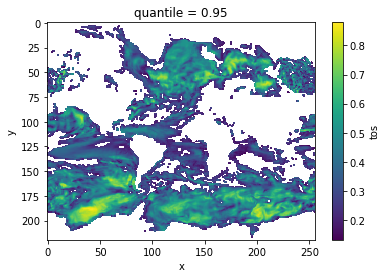## Decorrelation time¶

Taking the lagged correlation further over all lags, the decorrelation time shows the time after which the autocorrelation fell beyond its e-folding [vonStorch 1999]

:

threshold = climpred.bootstrap._bootstrap_func(climpred.stats.decorrelation_time,control3d,'time',bootstrap=100)
decorr_time = climpred.stats.decorrelation_time(control3d)
decorr_time.where(decorr_time>threshold).plot(yincrease=False, robust=False)

:

<matplotlib.collections.QuadMesh at 0x7fa3312afa58>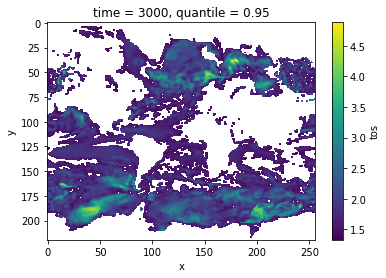## Verify diagnostic potential predictability in predictability simulations¶

Do we find predictability in the areas highlighted above also in perfect-model experiments?

:

ds3d = climpred.tutorial.load_dataset('MPI-PM-DP-3D')[varname].load()

:

bootstrap_skill = climpred.bootstrap.bootstrap_perfect_model(ds3d,
control3d,
metric='rmse',
comparison='m2e',
bootstrap=20)



:

init_skill = bootstrap_skill.sel(results='skill',kind='init')
# p value: probability that random uninitialized forecasts perform better than initialized
p = bootstrap_skill.sel(results='p',kind='uninit')

:

init_skill.where(p<=.05).plot(col='lead', robust=True, yincrease=False)

:

<xarray.plot.facetgrid.FacetGrid at 0x7fa311a2c898>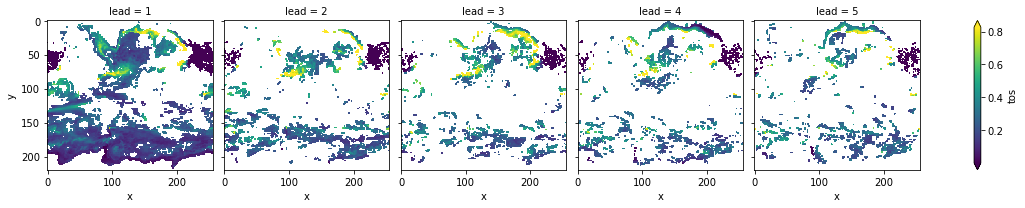The metric rmse is negatively oriented, e.g. higher values show large disprepancy between members and hence less skill.

As suggested by DPP, the variance-weighted mean period and autocorrelation, also in slight perturbed initial values ensembles there is predictability in the North Atlantic, North Pacific and Southern Ocean in sea-surface temperatures.